Courses

# Origin Of Quantum Mechanics (MSQ)

## 10 Questions MCQ Test Topic wise Tests for IIT JAM Physics | Origin Of Quantum Mechanics (MSQ)

Description
This mock test of Origin Of Quantum Mechanics (MSQ) for Physics helps you for every Physics entrance exam. This contains 10 Multiple Choice Questions for Physics Origin Of Quantum Mechanics (MSQ) (mcq) to study with solutions a complete question bank. The solved questions answers in this Origin Of Quantum Mechanics (MSQ) quiz give you a good mix of easy questions and tough questions. Physics students definitely take this Origin Of Quantum Mechanics (MSQ) exercise for a better result in the exam. You can find other Origin Of Quantum Mechanics (MSQ) extra questions, long questions & short questions for Physics on EduRev as well by searching above.
*Multiple options can be correct
QUESTION: 1

### The dispersion law for a certain type of wave motion is ω = (c2k2 + m2)1/2  where ω is the angular frequency, k is the magnitude of the propagation vector and c, m are constants. The group velocity of these waves approaches :

Solution: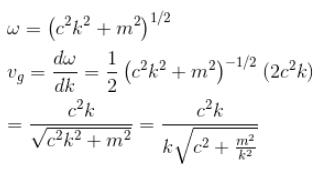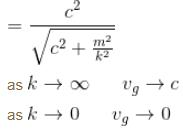*Multiple options can be correct
QUESTION: 2

### In the photoelectric effect experiment we use a source of light whose frequency can be varied. If we gradually increase the frequency of the incident light then :

Solution:

No photocurrent till the incident frequency reaches the minimum frequency required to overcome the work function of the metal φ

i.e. hvin = φ

After that, any increase in the frequency will not increase the photocurrent because the incident light has the same intensity.

Whereas KEmax increase linearly with the frequency of the incident radiation.

The correct answers are: Photoelectric effect observed (after some time) with photocurrent remaining constant., Photoelectric effect observed after some time with the maximum kinetic energy (KE) of electron varying (increasing with time)

*Multiple options can be correct
QUESTION: 3

### In case of an one electron Bohr atom, pn is the linear momentum of the electron in the nth orbit and En is its total energy. Which of the following is true? Here rn is the radius of the nth orbit and m is the mass.

Solution:

From the quantization of orbital angular momentum,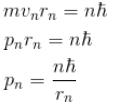For energy,
Centrifugal force = Coulomb attraction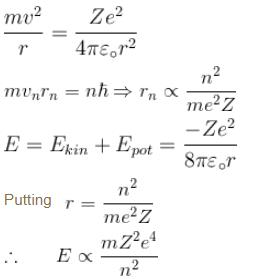The correct answers are: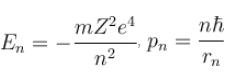*Multiple options can be correct
QUESTION: 4

For single electron atom with orbital angular momentumwhich of the following can be the possible values of a measurement of Lz. (z  component of L)?

Solution: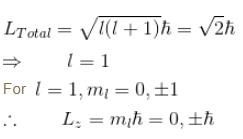The correct answers are: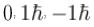*Multiple options can be correct
QUESTION: 5

For the photoelectric effect, stopping potential v/s incident frequency is plotted. Choose the correct statements.

Solution:

For photoelectric effect,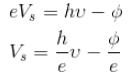Compare with y = mx + c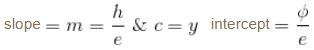The correct answers are: the graph is a straight line with a positive slope and negative y intercept., slope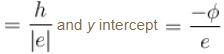*Multiple options can be correct
QUESTION: 6

The kinetic energy of electrons ejected from the surface of a metal ranges from :

Solution:

KEmin = 0 when the incident energy is just equal to the work function.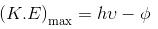⇒  when all the excess energy is given to the ejected electron and no energy is lost.

The correct answer is: 0 to  hv - φ

*Multiple options can be correct
QUESTION: 7

Which of the following is true.

Solution:

The correct answers are: Interference and Diffraction are manifestations of wave nature of light., Photoelectric and  compton effects are manifestations of particle nature of light.

*Multiple options can be correct
QUESTION: 8

In compton effect,

Solution:

Refer the theory of compton effect λ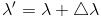The correct answers are: The scattered radiation contains X-rays of the incident frequency and frequency smaller than the incident frequency (vin), The scattered radiation contains X-rays of wavelength same as that of incident wavelength and larger than the incident wavelength

*Multiple options can be correct
QUESTION: 9

Which of the following is true about the absorption and emission of energy by an atom?

Solution:

E = hv Ef – Ein

The frequency can only corresponds to the difference in two energy states.
The correct answers are: An atom can only absorb photons that have certain specific energies., An atom can emit photons of only certain specific energies., At low temperatures, the lines in the absorption spectrum of an atom coincide with the lines in its emission spectrum that represent transitions to the ground slate

*Multiple options can be correct
QUESTION: 10

A singly ionized Helium atom in n = 4 state emits a photon of wavelength 470nm. If Ef is the final state energy and nf is the principal quantum number of the final state then :

Solution: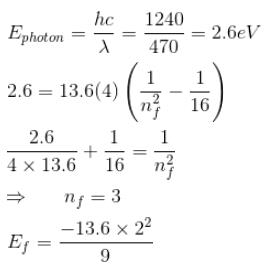= –6.0 eV
The correct answers are: Ef = –6.0 eVnf = 3